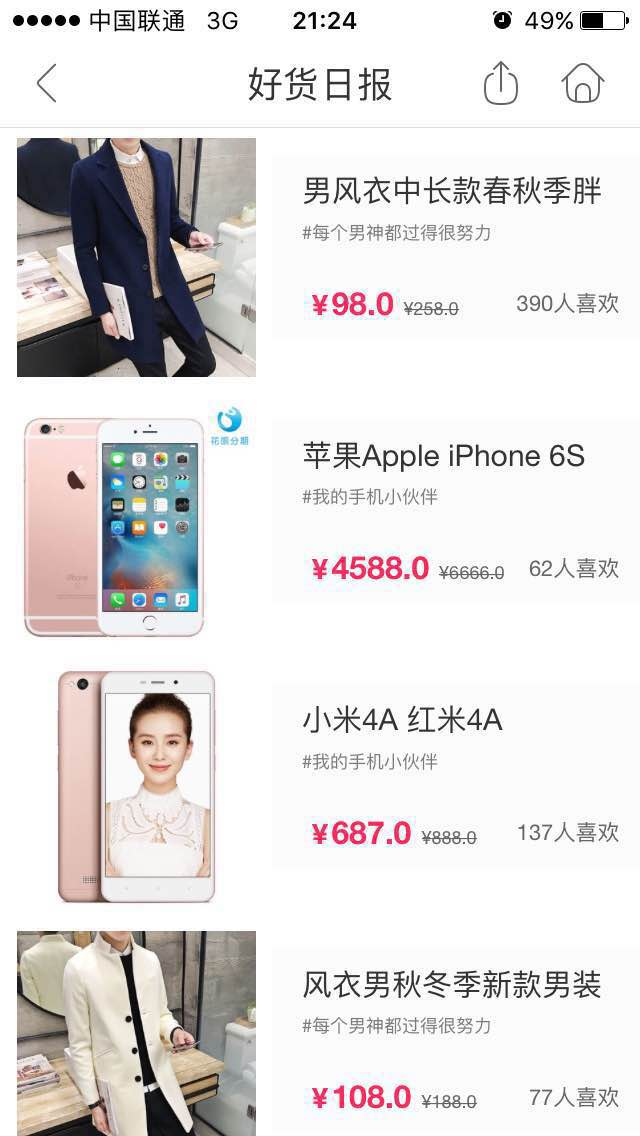## 问题背景## 基于User Profile的商品推荐

• 分析用户的类目偏好；
• 建立兴趣商品池。

### 用户类目偏好

• 女装
• 裙子、半身群、衬衫、T恤、西装外套、羽绒服、风衣、连衣裙、马甲
• 男装
• Polo衫、爸爸装、裤装、卫衣、工装制服
• 零食
• 酒水/茶/冲饮、坚果/特产、保健食品
• 3C数码
• 台式机/网络设备、平板/笔记本电脑、数码相机、单反相机
• 家具百货
• 床上用品、收纳整理、办公用品、文具用品

$score=(s^{click}, s^{fav}, s^{cart}, s^{order}).$

$score^{click}_{n}(U, I)=\alpha^{n}\cdot s^{(U, I, click)}$

$count_{n}(U, I)=(cnt^{(U, I, click)}_{n}, cnt^{(U, I, fav)}_{n}, cnt^{(U, I, cart)}_{n}, cnt^{(U, I, order)}_{n}).$

$score_{n}(U, I)=\sum_{b \in \{click, fav, cart, order\}}(\alpha^{n}\cdot s^{(U, I, b)}\cdot cnt^{(U, I, b)}_{n}).$

• $(I_{1}, I_{2}, \cdots, I_{m})$

$$\begin{eqnarray} score(U, C) & = & \sum_{j=1}^{t}\sum_{i=1}^{m}score_{j}(U, I_{i}) \\ {} & = & \sum_{j=1}^{t}\sum_{i=1}^{m}\sum_{b \in \{click, fav, cart, order\}}(\alpha^{j}\cdot s^{(U, I_{i}, b)}\cdot cnt^{(U, I_{i}, b)}_{j}). \end{eqnarray}$$

### 用户类目多样化

• 当用户行为比较稀疏，或者用户兴趣非常集中时，寻找到的用户兴趣个数非常少，如1个、2个。这时推给用户的列表商品比较单一；
• 用户对直接有过行为的商品的类目有兴趣，按兴趣类目推荐出来的商品惊喜度不够。

• $\{(I_{1}^{C_{i}}, I_{1}^{C_j}, sim_{cosine}(I_{1}^{C_{i}}, I_{1}^{C_j}), (I_{2}^{C_{i}}, I_{2}^{C_j}, sim_{cosine}(I_{2}^{C_{i}}, I_{2}^{C_j}),\cdots,(I_{n}^{C_{i}}, I_{n}^{C_j}, sim_{cosine}(I_{n}^{C_{i}}, I_{n}^{C_j})).$

$$sim(C_{i}, C_{j})=\sum_{t=1}^{n}sim_{cosine}(I_{t}^{C_{i}}, I_{t}^{C_{j}}).$$

### 建立兴趣商品池

• 各类目下的热销商品；
• 根据各类目种子商品寻找相似商品。